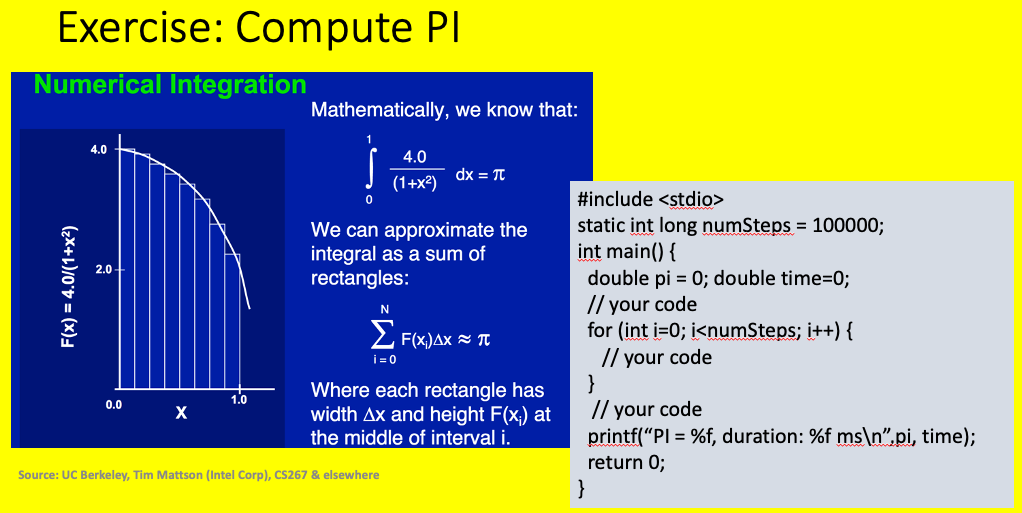# C: Assignments Day 1¶

Today we have three problems for you to tackle. Two are familiar and you performed them in Python as part of day 1 exercises and assignments. The third pi is new.

Warning

In the Python part of the bootcamp pretty much everything was set up for you with Jupyter notebooks that were supplied. In this part of the bootcamp we will only be providing you with ONE example file. You will in a hurry have to learn some emacs and basic git and linux commands. The sidebar on the left contains some basic instruction on these (enough to get you through this part of the bootcamp). The training wheels have been removed!

## Problem 1: Solve the Quadratic¶

We wish to solve the quadratic equations, i.e. given a, b, and c, solve the following equation for x.

$ax^2+bx+c=0$

The solution from your high school days is the legendary formula:

$x = \frac{-b \pm \sqrt{b^2 - 4ac}}{2a}$

We want you to write an application developed in C to solve this equation.

To start you off, we have provided a file solveQuadratic.c in /code/c/ExerciseDay1/ which will read 3 numbers from the command line and print out a message to the screen. You are to modify it to print out the solution for x. The contents of this file are shown below.

 1// program to solve quadratic equation
2//        ax^2 + bx + c = 0
3//
4// soln: x = -b/2a +/- sqrt(b^2-4ac)/2a
5//
6// write a program to take 3 inputs and output the soln
7// deal with possible errors in input, i.e. b^2-4ac negative
8
9#include <stdlib.h>
10#include <stdio.h>
11#include <math.h>
12
13int main(int argc, char **argv) {
14
15  if (argc != 4) {
16    printf("Usage: appName a b c\n");
17    exit(-1);
18  }
19
20  float a = atof(argv);
21  float b = atof(argv);
22  float c = atof(argv);
23
24  printf("Have a Nice Day!\n");
25  return 0;
26}
27


Note

When compiling because you will be using functions from the C math library you will need to include the math library when you compile and link your code, i.e.

gcc solveQuadratic.c -lm


## Problem 2: Stress Transformations¶

To transform stress to a rotated coordinate system one can use the formula shown in the figure. We would ask you to write code that will take as input 4 values sigmaXX, sigmaYY, tauXY, and theta compute the stress in the transformed coordinate system. We would ask you to perform that transformation computation in a function other than main and to complicate things, but demonstrate you understand passing of arrays, pass the input and output stresses to this new function in fixed length arrays.

Theory: Stress transformation

$\begin{split}\sigma_x' = \sigma_x \cos^2\theta + \sigma_y \sin^2\theta + 2 \tau_{xy} \sin\theta \cos\theta \\ \sigma_y' = \sigma_x \sin^2\theta + \sigma_y \cos^2\theta - 2 \tau_{xy} \sin\theta \cos\theta \\ \tau_{xy}' = ( \sigma_y - \sigma_x ) \sin\theta \cos\theta + \tau_{xy} ( \cos^2\theta - \sin^2\theta )\end{split}$

Given state:

$\sigma_x = 12~\text{ksi}~, \qquad \sigma_y = -5.5~\text{ksi}~, \qquad \tau_{xy} = 3.5~\text{ksi}$

Note

If you need something extra work, write to a file the results of this transformation from 0 through 360 degrees in increments you input from the command line.

To send you data to a file named results.out, start the application as follows:

./appName 1.0 100.0 0.01 1 > results.out

### Problem 3: Compute PI numerically¶

The figure below shows an method to compute pi by numerical integration. We would like you to implement that computation in a C program.Computation of pi numerically

Note

You will be using your solution on Day 4 as part of the parallel exercises.